Greg Petrics

•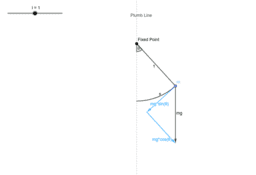Developing the Second Order Differential Equation that Models Simple Pendular Motion

Activity

Greg Petrics

•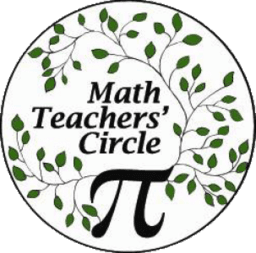Tessellations: Techniques and Teaching

Book

Greg Petrics

•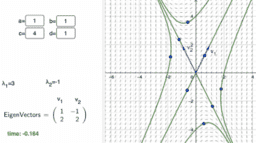Differential Equations For The People

Book

Greg Petrics

•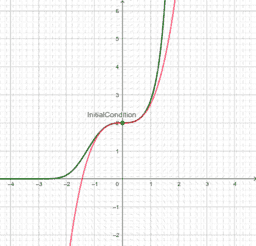Picard's Method of Successive Approximations Calculator

Activity

Greg Petrics

•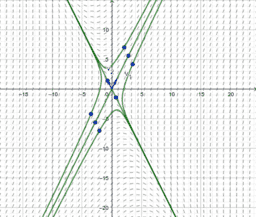Phase Plane Sketcher

Activity

Greg Petrics

•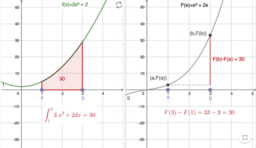Calculus For The People

Book

Greg Petrics

•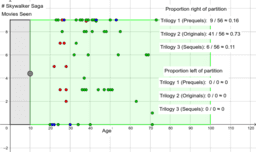The Recursive Partitioning Supervised Machine Learning Algorithm

Activity

Greg Petrics

•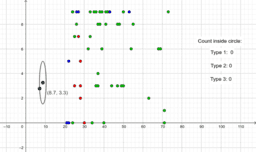The knn Supervised Machine Learning Algorithm

Activity

Greg Petrics

•DEPRECATED knn Classification

Activity

Greg Petrics

•The Pythagorean Theorem and the Law of Cosines

Activity

Greg Petrics

•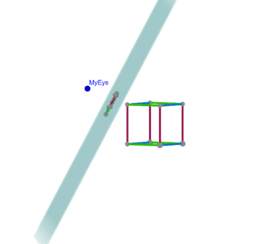Understanding Multiple Vanishing Points

Activity

Greg Petrics

•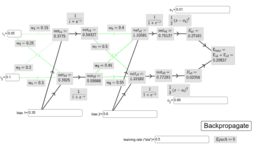Artificial Neural Network Example

Activity

Greg Petrics

•Circle Packing and Room Capacities for COVID 19

Activity

Greg Petrics

•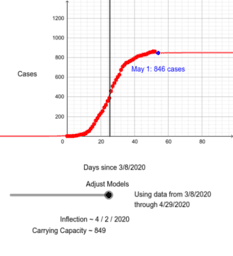Logistic Models of COVID19 in Vermont Over Time

Activity

Greg Petrics

•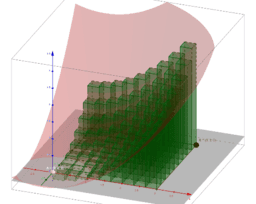Visualizing Riemann Sums of a Double Iterated Integral

Activity

Greg Petrics

•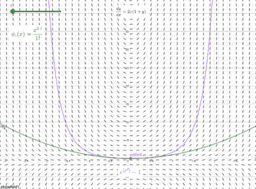Picard's Method of Successive Approximations

Activity

Greg Petrics

•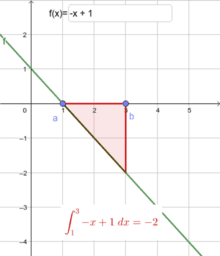The Fundamental Theorem of Calculus

Activity

Greg Petrics

•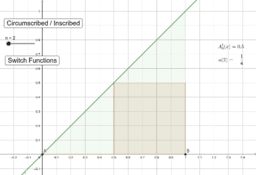Estimating Area under Functions (VMI Fall 2019)

Activity

Greg Petrics

•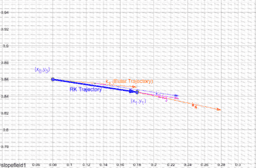Runge Kutta 4

Activity

Greg Petrics

•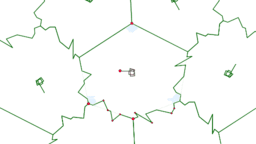Pseudo-Regular Hexagonal Tessellation Generator

Activity

Greg Petrics

•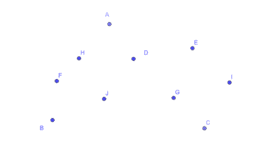Straighten it Out -- MMTC

Book

Greg Petrics

•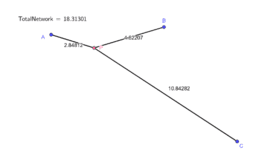Fermat Point of a Triangle

Activity

Greg Petrics

•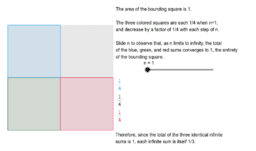Archimedes' Infinite Sum

Activity

Greg Petrics

•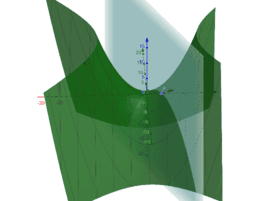Activity

Greg Petrics